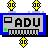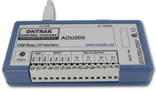ADU Command Summary The ADU interfaces operate using simple ASCII commands. Following is a list of commands for the ADU200 product.ADU200 RELAY COMMANDS ( PORT K ) SKn             Sets relay specified by n ( n = 0 ,1,2 or 3  ) RKn             Resets relay specified by n ( n= 0 , 1, 2 or 3 ) MKdd          Sets PORTK to decimal value dd  ( dd= 0 to 15 ) SPKxxxx     Sets PORT K to binary value xxxx  ( x= 0 or 1 ) RPKn          Returns status of relay specified by n ( n= 0 , 1, 2 or 3 ) RPK             Returns status of PORT K in binary format. PK                Returns status of PORT K in decimal format. DIGITAL COMMANDS ( PORT A ) RPAn           Returns status of Input   specified by n ( n= 0 , 1, 2 or 3 ) RPA              Returns status of PORT A in binary format. PA                 Returns status of PORT A in decimal format. EVENT COUNTER COMMAND SUMMARY REx                Returns present count of event counter ( x = 0,1,2 3 or H ) RCx                Returns present count and clears event counter ( x = 0,1,2, 3 or H  ) DBn                Sets de-bounce time of event counters ( n=0,1,2 or 3 ) ( 0 =10ms, 1 = 1ms, 2  = 100us   ) DB                Returns present de-dounce setting. SPECIAL FEATURE COMMAND SUMMARY SBn                Sets baud rate of auxillary RS232 port. (n = 0,1,2 or 3  ) ( 0 =9600, 1 = 19.2K, 2 =38K,  3 = 56K   ) SB                   Returns present baud rate setting. WDn                Sets watchdog timeout length (n = 0,1,2 or 3  ) ( 0 =WD OFF , 1 = 1s, 2 =10s,  3 = 1min.   ) WD                   Returns watchdog setting.